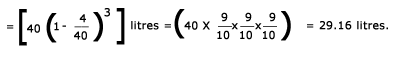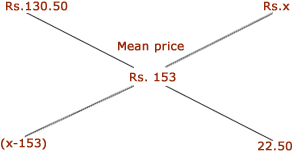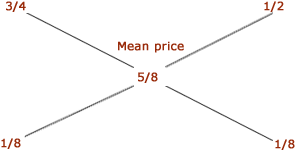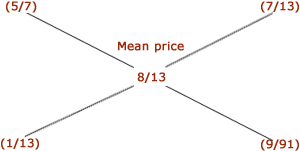## Arithmatical – Alligation or Mixture

DIRECTIONS : Important Facts and Formulae
1. A container contains 40 litres of milk. From this container 4 litres of milk was taken out and replaced by water. This process was repeated further two times. How much milk is now contained by the container. A.  26.34 litres B.  27.36 liters C.  28 litres D.  29.16 litres
Solution
 Amount of milk left after 3 operations2. Tea worth Rs.126 per kg are mixed with a third variety in the ratio 1: 1 : 2. If the mixture is worth Rs.153 per kg, the price of the third variety per kg will be A.  Rs.169.50 B.  Rs.1700 C.  Rs. 175.50 D.  Rs. 180
Solution
 Since first second varieties are mixed in equal proportions, so their average price = Rs.(126+135/2) = Rs.130.50 So, the mixture is formed by mixing two varieties, one at Rs. 130.50 per kg and the other at say, Rs. x per kg in the ratio 2 : 2, i.e., 1 : 1. We have to find x. Cost of 1 kg tea of 1st kind Cost of 1 kg tea of 2nd kindx-153/22.50 = 1 =› x - 153 = 22.50 =› x=175.50. Hence, price of the third variety = Rs.175.50 per kg.

3. A milk vendor has 2 cans of milk. The first contains 25% water and the rest milk. The second contains 50% water. How much milk should he mix from each of the containers so as to get 12 litres of milk such that the ratio of water to milk is 3 : 5? A.  4 litres, 8 litres B.  6 litres, 6 litres C.  5 litres, 7 litres D.  7 litres, 4 litres
Solution
 Let the cost of 1 litre milk be Re. 1 Milk in 1 litre mix. in 1st can = 3/4 litre, C.P. of 1 litre mix. in 1st can Re. 3/4 Milk in 1 litre mix. in 2nd can = 1/2 litre, C.P. of 1 litre mix. in 2nd can Re. 1/2 Milk in 1 litre of final mix. = 5/8 litre, mean price = Re. 5/8. By the rule of alligation, we have: Cost of 1 kg mixture of 1st kind Cost of 1 kg mixture of 2nd kindRatio of two mixtures = 1/8 : 1/8 = 1:1. So, quantity of mixture taken from each can = (1/2 X 12) = 6 litres.
4. Two vessels A and B contain spirit and water in the ratio 5 : 2 and 7 : 6 respectively. Find the ratio in which these mixture be mixed to obtain a new mixture in vessel C containing spirit and water in the ration 8 : 5? A.  4 : 3 B.  3 : 4 C.  5 : 6 D.  7 : 9
Solution
 Let the C.P. of spirit be Re. 1 litre. Spirit in 1 litre mix. of A = 5/7 litre, C.P. of 1 litre mix. in A = Re. 5/7 Spirit in 1 litre mix. of B = 7/13 litre, C.P. of 1 litre mix. in B = Re. 7/13 Spirit in 1 litre mix. of C = 8/13 litre, Mean price = Re. 8/13. By the rule of alligation, we have: Cost of 1 litre mixture in A Cost of 1 litre mixture in BRequired ratio = 1/13 : 9/91 = 7 : 9.
5. A can contains a mixture of two liquids A and B in the ratio 7 : 5. When 9 litres of mixture are drawn off and the can is filled with B, the ratio of A and B becomes 7 : 9. How many litres of liquid A was contained by the can initially? A.  10 B.  20 C.  21 D.  25
Solution
Suppose the can initially contains 7x and 5x litres of mixtures A and B respectively
Quantity of A in mixture left
= (7x - 7/12 x 9) litres = (7x - 21/4) litres.
Quantity of B in mixture left
= (5x - 5/12 x 9) litres = (5x - 15/4) litres.
(7x - 21/4) / [(5x - 15/4)+9] = 7/9 = › 28x - 21/20x + 21 = 7/9 =› 252x - 189 = 140x + 147
=› 112x = 336 =’ x = 3.
So, the can contained 21 litres of A.
Page 1 of 4 1234